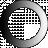# American Dictionary of the English Language

Dictionary Search

### Proportion

PROPORTION, noun [Latin proportio; pro and portio, part or share. See Portion.]

1. The comparative relation of any one thing to another. Let a man's exertions be in proportion to his strength.

2. The identity or similitude of two ratios. proportion differs from ratio. Ratio is the relation which determines the quantity of one thing from the quantity of another, without the intervention of a third. Thus the ratio of 5 and Isaiah 2:1; the ratio of 8 and 16 is 2. proportion is the sameness or likeness of two such relations. Thus 5 is to 10, as 8 to 16, or A is to B, as C is to D; that is, 5 bears the same relation to 10, as 8 does to 16. Hence we say, such numbers are in proportion

PROPORTION, in mathematics, an equality or ratios.

The term proportion is sometimes improperly used for ratio. The ratio between two quantities, is expressed by the quotient of one divided by the other; thus, the ratio of 10 to 5 is 2, and the ratio of 16 to 8 is 2. These two equal ratios constitute a proportion which is expressed by saying, 10 is to 5 as 16 is to 8; or more concisely, 10:5::16:8. [See Ratio.]

3. In arithmetic, a rule by which, when three numbers are given, a fourth number is found, which bears the same relation to the third as the second does to the first; or a fourth number is found, bearing the same relation to the second as the first does to the third. The former is called direct, and the latter, inverse proportion

4. Symmetry; suitable adaptation of one part or thing to another; as the proportion of one limb to another in the human body; the proportion of the length and breadth of a room to its highth.

Harmony, with every grace,

Place in the fair proportions of her face.

5. Equal or just share; as, to ascertain the proportion of profit to which each partner in a company is entitled.

6. Form; size. [Little Used.]

7. The relation between unequal things of the same kind, by which their several parts correspond to each other with an equal augmentation and diminution, as in reducing and enlarging figures.

[This more properly belongs to ratio.]

Harmonical or musical proportion is when, of three numbers, the first is to the third as the difference of the first and second to the difference of the second and third. Thus 2.3.6. are in harmonical proportion; for 2 is to 6 as 1 to 3. So also four numbers are harmonical, when the first is to the fourth, as the difference of the first and second is to the difference of the third and fourth. Thus, 24.16.12.9. are harmonical, for 24 :9 ::8 :3.

Arithmetical and geometrical proportion [See Progression, No.4.]

Reciprocal proportion an equality between a direct and a reciprocal ratio. Thus, 4 :2 ::1/3 :1/6. [See Reciprocals, and Reciprocal ratio.]

PROPORTION, verb transitive To adjust the comparative relation of one thing or one part to another; as, to proportion the size of a building to its highth, or the thickness of a thing to its length; to proportion our expenditures to our income.

In the loss of an object, we do not proportion our grief to its real value, but to the value our fancies set upon it.

1. To form with symmetry or suitableness, as the parts of the body.